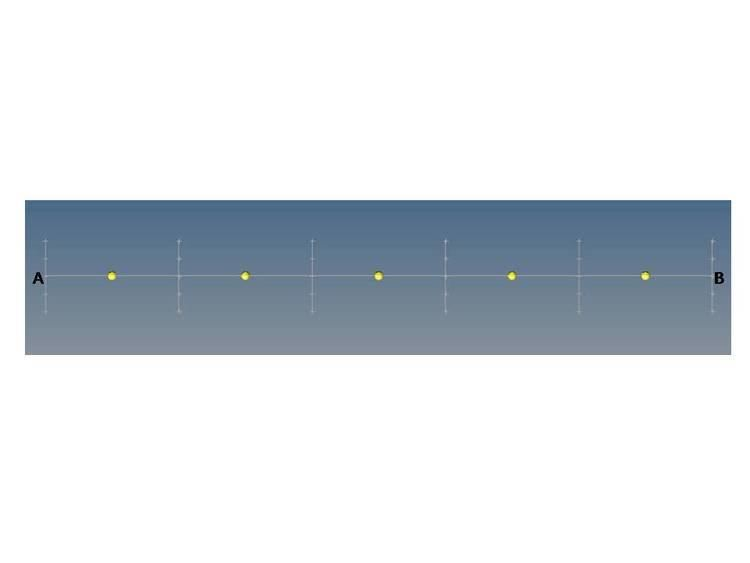# Finite volume method for one dimensional steady state diffusion

Updated on
Covid-19Finite volume method in computational fluid dynamics is a discretization technique for partial differential equations that arise from physical conservation laws. These equations can be different in nature, e.g. elliptic, parabolic, or hyperbolic. First well-documented use was by Evans and Harlow (1957) at Los Alamos. The general equation for steady diffusion can be easily be derived from the general transport equation for property Φ by deleting transient and convective terms.

General Transport equation can be define as

ρ ϕ t + div ( ρ ϕ υ ) = div ( Γ grad ϕ ) + S ϕ

where,
ρ is density and ϕ is conservative form of all fluid flow,
Γ is the Diffusion coefficient and S is the Source term.
div ( ρ ϕ υ ) is Net rate of flow of ϕ out of fluid element(convection),
div ( Γ grad ϕ ) is Rate of increase of ϕ due to diffusion,
S ϕ is Rate of increase of ϕ due to sources.

ρ ϕ t is Rate of increase of ϕ of fluid element(transient),

Conditions under which the transient and convective terms goes to zero:

• Steady State
• Low Reynolds Number
• For one-dimensional steady state diffusion, General Transport equation reduces to:

or,

The following steps comprehend one-dimensional steady state diffusion -

STEP 1
Grid Generation

• Divide the domain in equal parts of small domain.
• Place nodal points midway in between each small domain.
• Create control volume using these nodal points.
• Create control volume near the edge in such a way that the physical boundaries coincide with control volume boundaries.(Figure 1)
• Assume a general nodal point 'P' for a general control volume.Adjacent nodal points in east and west are identified by E and W respectively.The west side face of the control volume is referred to by 'w' and east side control volume face by 'e'.(Figure 2)
• The distance between WP, wP, Pe and PE are identified by δ x W P , δ x w P , δ x P e and δ x P E respectively.(Figure 4)
• STEP 2
Discretization

• The crux of Finite volume method is to integrate governing equation all over control volume, known discretization.
• Nodal points used to discretize equations.
• At nodal point P control volume is defined as (Figure 3)

• Δ V d d x ( Γ d ϕ d x ) d V + Δ V S d V = ( Γ A d ϕ d x ) e ( Γ A d ϕ d x ) w + S Δ V = 0

where

A is Cross-sectional Area Cross section (geometry) of control volume face, Δ V is Volume, S is average value of source S over control volume

• It states that diffusive flux Fick's laws of diffusion ϕ from east face minus west face leads to generation of flux in control volume.
• ϕ diffusive coefficient and d ϕ d x is required in order to interpreter useful conclusion.
• Central differencing technique  is used to derive ϕ diffusive coefficient.

• Γ w = Γ W + Γ P 2

Γ w = Γ P + Γ E 2

• d ϕ d x gradient from east to west is calculated with help of nodal points.(Figure 4)
• ( d ϕ d x ) e = ϕ E ϕ P δ x P E
( d ϕ d x ) w = ϕ P ϕ W δ x W P
• In practical situation source term can be linearize

• S Δ V = S u + S p ϕ p

• Merging above equations leads to
• Γ e A e ( ϕ E ϕ P δ x P E ) Γ w A w ( ϕ P ϕ W δ x P E ) + ( S u + S p ϕ p )

• Re-arranging

• ( Γ e δ x P E A e + Γ w δ x W P A w S p ) ϕ P = ( Γ w δ x W P A w ) ϕ W + ( Γ e δ x W P A e ) ϕ E + S u

• Compare and identify above equation with

• a P ϕ P = a W ϕ W + a E ϕ E + S u

where

STEP 3:
Solution of equations

• Discretized equation must be set up at each of the nodal points in order to solve the problem.
• The resulting system of linear algebraic equation Linear equation is then solved to obtain distribution of the property ϕ at the nodal points by any form of matrix solution technique.
• The matrix of higher order  can be solved in MATLAB.
• This method can also be applied to a 2D situation. See Finite volume method for two dimensional diffusion problem.

## References

Topics

 B i Link H2
 L